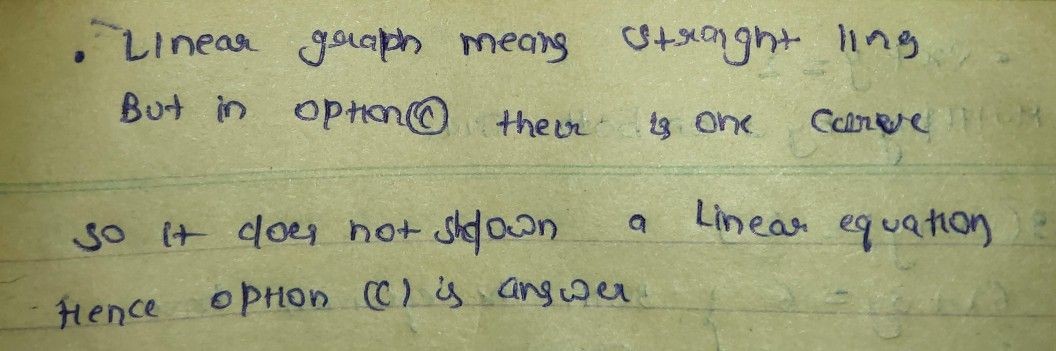Symbol
Problem$1$ Which of the following graph does not show an example of system of linear equation in two variables? $D\right)$ A) B) $C\right)$ $0$